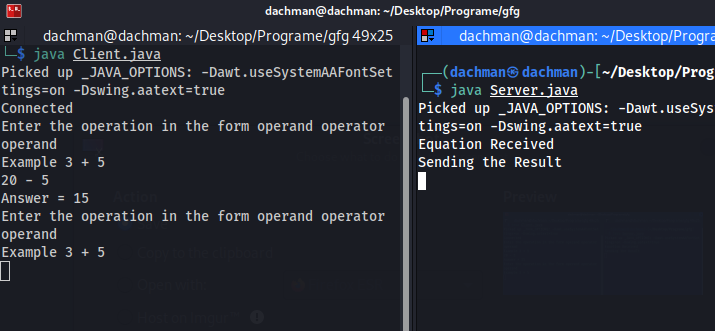# Simple Calculator using Java Socket Programming

• Last Updated : 02 Jun, 2022

Prerequisite: Socket Programming in Java

First, we understand the basics of java socket programming. Java Socket is used to communicate between two different JREs. Java socket can be connection-oriented or connection-less. In java, we have a package called “java.net”. In this package, we have two classes Socket Class and Server Class. Those classes are used to create connection-oriented or connection-less programs. In this article, We will see how the java server will perform the basic operations and send back the result to the java client

### Java Client

First, we create and write client-side socket code. In client socket program must know two information

• IP Address of Server and,
• Port Number

## Java

 `// A Java program for a Client` `import` `java.io.*;` `import` `java.net.*;` `import` `java.util.*;`   `public` `class` `Client {` `    ``private` `Socket s = ``null``;` `    ``public` `Client(String address, ``int` `port)` `    ``{` `        ``try` `{` `            ``// In this Code Input Getting from a user` `            ``Scanner sc = ``new` `Scanner(System.in);` `            ``s = ``new` `Socket(address, port);` `            ``System.out.println(``"Connected"``);`   `            ``// Create two objects first is dis and dos for` `            ``// input and output` `            ``DataInputStream dis` `                ``= ``new` `DataInputStream(s.getInputStream());` `            ``DataOutputStream dos` `                ``= ``new` `DataOutputStream(s.getOutputStream());`   `            ``// Making a Loop` `            ``while` `(``true``) {` `                ``System.out.println(` `                    ``"Enter the operation in the form operand operator operand"``);` `                ``System.out.println(``"Example 3 + 5 "``);` `                ``String inp = sc.nextLine();`   `                ``// Check the user input if user enter over` `                ``// then` `                ``// connect is stopped by server and user` `                ``if` `(inp.equals(``"Over"``))` `                    ``break``;` `                ``dos.writeUTF(inp);`   `                ``String ans = dis.readUTF();` `                ``System.out.println(``"Answer = "` `+ ans);` `            ``}` `        ``}` `        ``catch` `(Exception e) {` `            ``System.out.println(``"Error in Connection"``);` `        ``}` `    ``}`   `    ``public` `static` `void` `main(String args[])` `    ``{` `        ``// Connection With Server port 5000` `        ``Client client = ``new` `Client(``"127.0.0.1"``, ``5000``);` `    ``}` `}`

### Java Server

In this Java Server, we are performing simple calculations example addition, subtraction, etc. In this Code first, we receive input from the client-side. We pass this information to the “Stringtokenizer class” this class help to extract the exact “operand and operation” to perform the operation. when the result is ready java server sends it back to the result client

## Java

 `// A Java program for a Server` `import` `java.io.*;` `import` `java.net.*;` `import` `java.util.*;`   `public` `class` `Server {` `    ``// initialize socket and input stream` `    ``private` `Socket socket = ``null``;`   `    ``// constructor with port` `    ``public` `Server(``int` `port)` `    ``{` `        ``try` `{` `            ``// Making a ServerSocket  object` `            ``// for receiving Client Request` `            ``ServerSocket ss = ``new` `ServerSocket(port);` `            ``Socket s = ss.accept();`   `            ``// dis and dos object for receiving` `            ``// input from client send output to client` `            ``DataInputStream dis` `                ``= ``new` `DataInputStream(s.getInputStream());` `            ``DataOutputStream dos` `                ``= ``new` `DataOutputStream(s.getOutputStream());`   `            ``while` `(``true``) {` `                ``String input = dis.readUTF();` `                ``if` `(input.equals(``"bye"``))` `                    ``break``;` `                ``System.out.println(``"Equation Received"``);` `                ``int` `result = ``0``;`   `                ``StringTokenizer st` `                    ``= ``new` `StringTokenizer(input);` `                ``int` `oprnd1` `                    ``= Integer.parseInt(st.nextToken());` `                ``String operation = st.nextToken();` `                ``int` `oprnd2` `                    ``= Integer.parseInt(st.nextToken());`   `                ``// Calculator Operation Perform By Server` `                ``if` `(operation.equals(``"+"``)) {` `                    ``result = oprnd1 + oprnd2;` `                ``}` `                ``else` `if` `(operation.equals(``"-"``)) {` `                    ``result = oprnd1 - oprnd2;` `                ``}` `                ``else` `if` `(operation.equals(``"/"``)) {` `                    ``result = oprnd1 / oprnd2;` `                ``}` `                ``else` `if` `(operation.equals(``"*"``)) {` `                    ``result = oprnd1 * oprnd2;` `                ``}` `                ``System.out.println(``"Sending the Result"``);` `                ``dos.writeUTF(Integer.toString(result));` `            ``}` `        ``}` `        ``catch` `(Exception e) {` `            ``System.out.println(``"Error"``);` `        ``}` `    ``}`   `    ``public` `static` `void` `main(String args[])` `    ``{` `        ``// Server Object and set port number 5000` `        ``Server server = ``new` `Server(``5000``);` `    ``}` `}`

Output:My Personal Notes arrow_drop_up
Recommended Articles
Page :